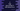# 3 different C programs to find the sum of first n odd natural numbers## C program to find the sum of first n odd natural numbers:

This post will show you how to find the sum of first n odd natural numbers in C. The program will take the value of n as the input from the user and print the sum.

A number is called odd if it is not divisible by 2. Before we start writing the program, let’s learn the algorithm to use in the program.

### Algorithm:

We have to use one loop to iterate from 1 to calculate the final sum.

We can use one loop and check for each value if it is an odd or even:

• Run one loop from 1.
• Initialize a sum variable as 0 to hold the final sum of all odd numbers. Initialize another variable as 0 to hold the total count of odd numbers found.
• For each value, check if it is odd or even. If it is odd, add it to the sum variable.
• If the count variable is equal to n, exit from the loop.
• Once the loop ends, print the value of the sum variable.

We can also run a loop from 1 with an increment of 2 on each iteration. This will skip all even numbers and it will iterate only the odd values. We can add all numbers it is iterating to get the final sum.

### Method 1: C program to find the sum of odd natural numbers by using a for loop:

Let’s write a program that uses a for loop to find the sum of first n odd numbers starting from 1. It will take the value of n as an input from the user.

``````#include <stdio.h>

int getOddSum(int n)
{
int sum = 0, count = 0;

for (int i = 1;; i++)
{
if (i % 2 != 0)
{
sum += i;
count++;
}
if (count == n)
break;
}
return sum;
}

int main()
{
int n;

printf("Enter the value value of n: ");
scanf("%d", &n);

printf("Sum of first %d odd numbers is: %d\n", n, getOddSum(n));
}``````

Here,

• The program is asking the user to enter the value of n. This value is stored in the variable n.
• It calls the getOddSum method to get the sum of the first n odd numbers starting from 1.
• It uses a for loop that runs from 1 . On each iteration, it checks the value of i. If it is odd, it adds it to the variable sum.
• It uses the count variable to hold the number odd variables added. Once it is equal to n, it breaks from the loop.
• It returns the value of sum at the end.

If you run this program, it will give outputs as below:

``````Enter the value value of n: 10
Sum of first 10 odd numbers is: 100

Enter the value value of n: 2
Sum of first 2 odd numbers is: 4``````

### Method 2: By incrementing 2 on each iteration:

We can change the above program to increment the value of i by 2 on each iteration. It will skip all even values and iterate only over the odd values.

``````#include <stdio.h>

int getOddSum(int n)
{
int sum = 0, count = 0;

for (int i = 1; count < n; i += 2)
{
sum += i;
count++;
}
return sum;
}

int main()
{
int n;

printf("Enter the value value of n: ");
scanf("%d", &n);

printf("Sum of first %d odd numbers is: %d\n", n, getOddSum(n));
}``````

We don’t have to check if the current value of i is odd or even. We can add all the numbers in the loop. It will give similar results.

``````Enter the value value of n: 3
Sum of first 3 odd numbers is: 9``````

### Method 3: By using a while loop:

We can also use a while loop to write the same program. It will increment the value of i by 2 similar to the above example.

``````#include <stdio.h>

int getOddSum(int n)
{
int sum = 0, count = 1, i = 1;

while (count <= n)
{
sum += i;
i += 2;
count++;
}
return sum;
}

int main()
{
int n;

printf("Enter the value value of n: ");
scanf("%d", &n);

printf("Sum of first %d odd numbers is: %d\n", n, getOddSum(n));
}``````

It will print similar output.